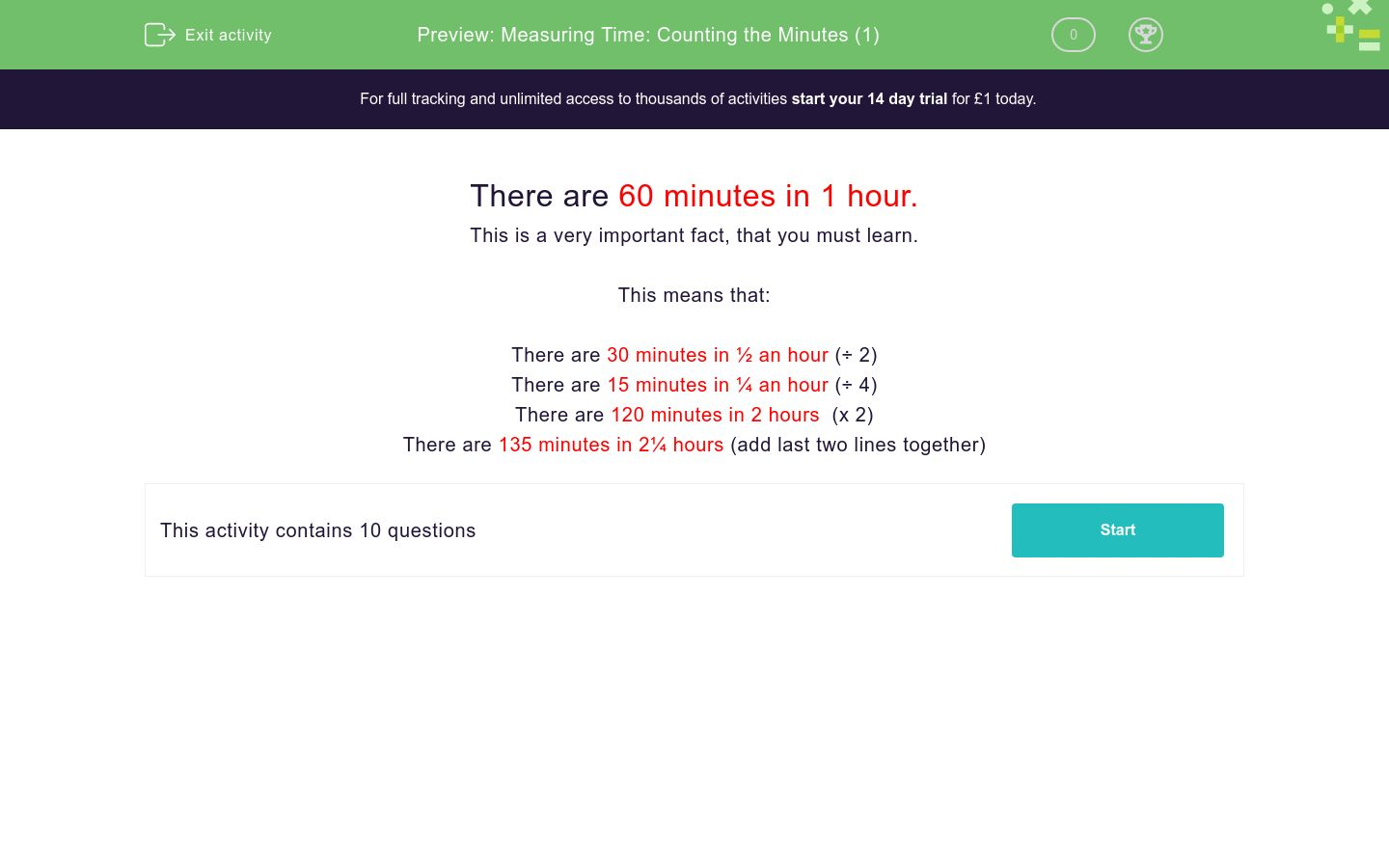# Measuring Time: Counting the Minutes (1)

In this worksheet, children must convert between hours and minutes.Key stage:  KS 1

Curriculum topic:   Measurement

Curriculum subtopic:   Know Divisions of Time

Difficulty level:### QUESTION 1 of 10

There are 60 minutes in 1 hour.

This is a very important fact, that you must learn.

This means that:

There are 30 minutes in ½ an hour (÷ 2)

There are 15 minutes in ¼ an hour (÷ 4)

There are 120 minutes in 2 hours (x 2)

There are 135 minutes in 2¼ hours (add last two lines together)

How many minutes are there in ½ hour?

(just write the number)

How many minutes are there in ¼ hour?

(just write the number)

How many minutes are there in 1½ hours?

(just write the number)

How many minutes are there in 1¼ hours?

(just write the number)

How many minutes are there in ¾ hour?

(just write the number)

How many hours are there in 120 minutes?

(just write the number)

How many hours are there in 15 minutes?

¼

½

¾

How many hours are there in 30 minutes?

¼

½

How many hours are there in 45 minutes?

½

¾

¼

How many hours are there in 105 minutes?

• Question 1

How many minutes are there in ½ hour?

(just write the number)

30
• Question 2

How many minutes are there in ¼ hour?

(just write the number)

15
• Question 3

How many minutes are there in 1½ hours?

(just write the number)

90
• Question 4

How many minutes are there in 1¼ hours?

(just write the number)

75
• Question 5

How many minutes are there in ¾ hour?

(just write the number)

45
• Question 6

How many hours are there in 120 minutes?

(just write the number)

2
• Question 7

How many hours are there in 15 minutes?

¼
• Question 8

How many hours are there in 30 minutes?

½
• Question 9

How many hours are there in 45 minutes?

¾
• Question 10

How many hours are there in 105 minutes?